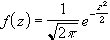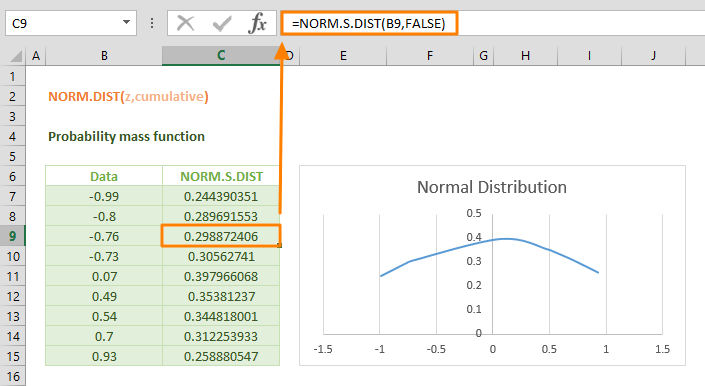The NORM.S.DIST is a Statistical function that calculates and returns the standard normal distribution of a specified value. The standard normal distribution is a normal distribution with a mean of zero (0) and a standard deviation of one (1). In this guide, we’re going to show you how to use the NORM.S.DIST function and also go over some tips and error handling methods.

## Supported versions

• Excel 2010 and later

## NORM.S.DIST (standard normal distribution) Function Syntax

NORM.DIST(z, cumulative)

## Arguments

 z The input value for which you want to calculate the distribution. cumulative A logical value which specifies the type of the distribution. FALSE = Probability density function TRUE = Cumulative distribution function

## NORM.S.DIST Examples

### Probability density function

The NORM.S.DIST function uses the probability density function when the cumulative argument is set to the FALSE logical value. The equation used for the normal density function iswhere z is the value for which you want the distribution result. For example, the standard normal density function returns approximately 0.29 for the value of -0.76.

=NORM.S.DIST(-0.76,FALSE)### Cumulative normal distribution function

The standard normal distribution function can also be used for calculating the cumulative normal distribution. The cumulative normal distribution function uses the integral from negative infinity to x of the probability density function. Set the cumulative argument to the TRUE logical value to evaluate its cumulative normal distribution.

=NORM.S.DIST(-0.76,TRUE)## Tips and Tricks

• The NORMSDIST is the older version of this function. We don't recommend using the NORMSDIST function.
• Other similar functions:
• DIST function returns the standard normal distribution for a specified mean and standard deviation.
• INV function returns the inverse of the cumulative normal distribution.
• S.INV function returns the inverse of the standard cumulative normal distribution.

## Common Issues

• The NORM.S.DIST function returns the #VALUE! error value, if the argument entered is non-numeric.